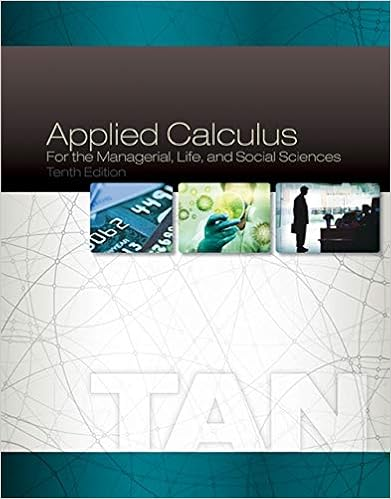# A what percentage of the total us income was received

• Notes
• mimi304
• 40
• 100% (1) 1 out of 1 people found this document helpful

This preview shows page 9 - 11 out of 40 pages.

##### We have textbook solutions for you!
The document you are viewing contains questions related to this textbook.The document you are viewing contains questions related to this textbook.
Chapter 6 / Exercise 2
Applied Calculus for the Managerial, Life, and Social Sciences
TanExpert Verified
(a) What percentage of the total US income was received by the richest 20% of the popu-lation in 2008? (b) Use a calculator or computer to fit a quadratic function to the data in the table. Graphthe data points and the quadratic function. Is the quadratic model a reasonable fit? (c) Use the quadratic model for the Lorenz function to estimate the Gini index for the United States in 2008. 3. The following table gives values for the Lorenz function in the years 1970, 1980, 1990,and 2000. Use the method of Problem 2 to estimate the Gini index for the United Statesfor those years and compare with your answer to Problem 2(c). Do you notice a trend? 4. A power model often provides a more accurate fit than a quadratic model for a Lorenz function. If you have a computer with Maple or Mathematica, fit a power function to the data in Problem 2 and use it to estimate the Gini index for the United States in 2008. Compare with your answer to parts (b) and (c) of Problem 2. G G G 2 y 1 0 x L x dx y x G y x y x y x y L x a % a % L x x 1, 1 0, 0 0.8, 0.5 12% 40% 0.4, 0.12 b % a % a 100, b 100 0, 1 y L x y ax k CAS FIGURE 1 0 1 0.8 0.6 0.4 0.2 y x (0.4, 0.12) 1 Lorenz curve for the US in 2008 (0.8, 0.5) FIGURE 2 0 1 y x (1, 1) y=x income fraction population fraction 1 FIGURE 3 0 1 y x y=x y=L(x) x 0.0 0.2 0.4 0.6 0.8 1.0 0.000 0.034 0.120 0.267 0.500 1.000 L x x 0.0 0.2 0.4 0.6 0.8 1.0 1970 0.000 0.041 0.149 0.323 0.568 1.000 1980 0.000 0.042 0.144 0.312 0.559 1.000 1990 0.000 0.038 0.134 0.293 0.530 1.000 2000 0.000 0.036 0.125 0.273 0.503 1.000
##### We have textbook solutions for you!
The document you are viewing contains questions related to this textbook.The document you are viewing contains questions related to this textbook.
Chapter 6 / Exercise 2
Applied Calculus for the Managerial, Life, and Social Sciences
TanExpert Verified
352 CHAPTER 5 In trying to find the volume of a solid we face the same type of problem as in finding areas. We have an intuitive idea of what volume means, but we must make this idea precise by using calculus to give an exact definition of volume. We start with a simple type of solid called a cylinder (or, more precisely, a right cylin- der ). As illustrated in Figure 1(a), a cylinder is bounded by a plane region , called the base , and a congruent region in a parallel plane. The cylinder consists of all points on line segments that are perpendicular to the base and join to . If the area of the base is and the height of the cylinder (the distance from to ) is , then the volume of the cylin- der is defined as In particular, if the base is a circle with radius , then the cylinder is a circular cylinder with volume [see Figure 1(b)], and if the base is a rectangle with length and width , then the cylinder is a rectangular box (also called a rectangular parallelepiped ) with volume [see Figure 1(c)]. For a solid S that isn’t a cylinder we first “cut” S into pieces and approximate each piece by a cylinder. We estimate the volume of S by adding the volumes of the cylinders. We arrive at the exact volume of S through a limiting process in which the number of pieces becomes large. We start by intersecting S with a plane and obtaining a plane region that is called a cross- section of Let be the area of the cross-section of in a plane perpendic- ular to the -axis and passing through the point , where . (See Figure 2. Think of slicing with a knife through and computing the area of this slice.) The cross-sectional area will vary as increases from to .
•••